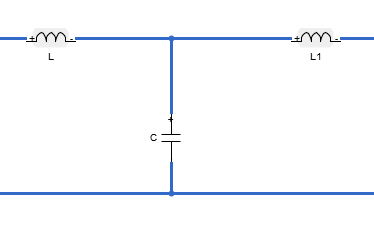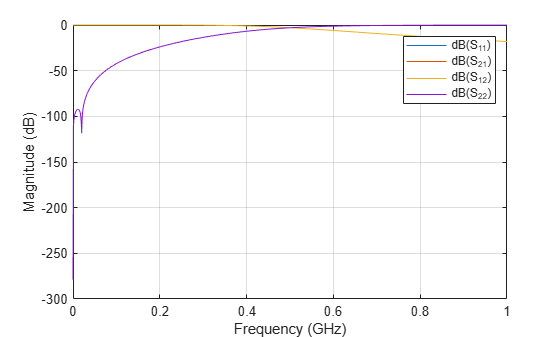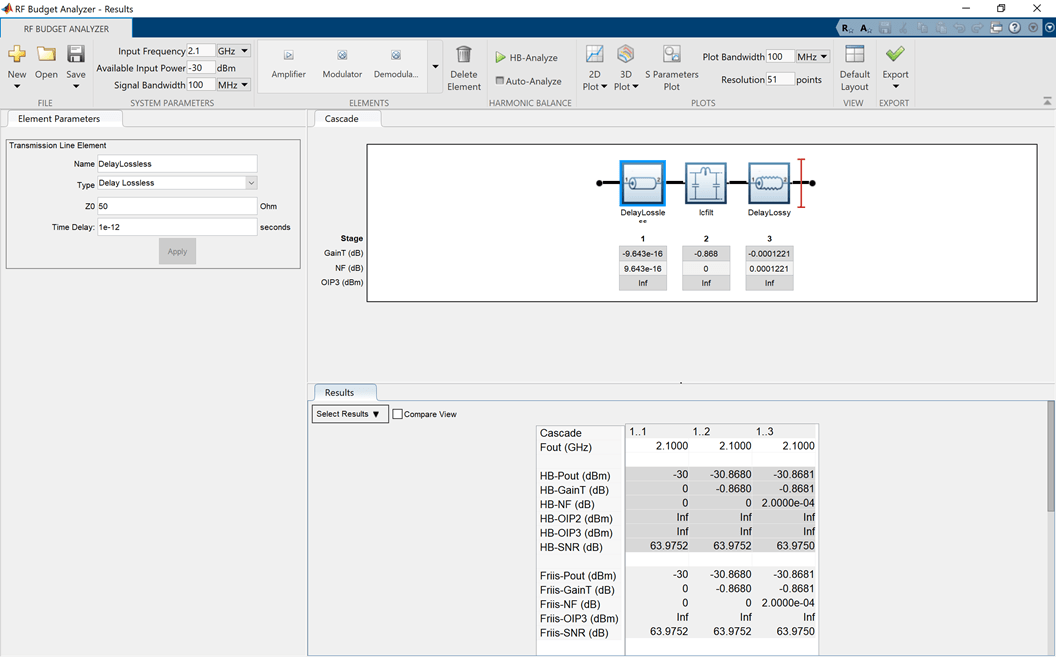## Description

Use an `lcladder` object:

• To create an LC ladder filter.

• To convert an `rffilter` object to an LC ladder.

• To model an LC circuit in cascaded stages using an `rfbudget` object or the RF Budget Analyzer app.## Creation

### Syntax

``lcobj = lcladder(top,L,C)``
``lcobj = lcladder(rffilterobj)``
``lcobj = lcladder(___,lcname)``

### Description

example

````lcobj = lcladder(top,L,C)` creates an LC ladder object `lcobj` and sets the Topology, Inductances, and Capacitances properties.```
````lcobj = lcladder(rffilterobj)` creates a LC ladder object `lcobj` from an RF filter object `rffilterobj`. ```
````lcobj = lcladder(___,lcname)` creates an LC ladder object `lcobj` and sets the Name property. Specify `lcname` after all other input arguments.```

### Input Arguments

expand all

RF filter object, specified as an `rffilter` object.

Example: ```lcobj = lcladder(rffilterobj)```

## Properties

expand all

Topology type of the LC ladder, specified as one of the following:

• `'lowpasspi'` — Low-pass pi filter

• `'lowpasstee'` —Low-pass tee filter

• `'highpasspi'` — High-pass pi filter

• `'highpasstee'` — High-pass tee filter

• `'bandpasspi'` — Band-pass pi filter

• `'bandpasstee'` — Band-pass tee filter

• `'bandstoppi'` — Band-stop pi filter

• `'bandstoptee'` — Band-stop tee filter

Example: `'lowpasspi'`

Inductor values in the LC ladder, specified as an `inductor` object or as a numeric scalar or two-element vector in henries.

Example: `1e-9`

Capacitor values in the LC ladder, specified as a `capacitor` object or as a numeric scalar or two-element vector in farads.

Example: `[2e-12 3e-12]`

Name of the LC ladder object, specified as a character vector or string scalar.

Example: `'lcfilter'`

Number of ports in LC ladder object. specified as a positive scalar.

Terminal names of LC ladder object, specified as the cell vector, `{'p1+' 'p2+' 'p1-' 'p2-'}`. An LC ladder object always has four terminals: two terminals associated with the first port (`'p1+'` and `'p1-'`) and two terminals associated with the second port (`'p2+'` and `'p2-'`).

Example: `{'p1+' 'p2+' 'p1-' 'p2-'}`

Parent circuit nodes connected to LC ladder object terminals, specified as a vector of integers. This property appears only after the LC ladder object is added to a circuit.

Note

`ParentNodes` are only displayed after the capacitor has been added into a circuit.

Full path of the circuit to which the LC ladder object belongs, specified as character vector. This path appears only after the inductor is added to the circuit.

Note

`ParentPath` is only displayed after the capacitor has been added into a circuit.

## Object Functions

 `sparameters` Calculate S-parameters for RF data, network, circuit, and matching network objects `groupdelay` Group delay of S-parameter object or RF filter object or RF Toolbox circuit object `clone` Create copy of existing circuit element or circuit object `rfplot` Plot S-parameter data

## Examples

collapse all

Create a low-pass pi LC ladder object with an inductor value of 3.18e-8 H and a capacitor value of 6.37e-12 F. Calculate and plot the s-parameters.

```L = 3.18e-8; C = [6.37e-12 6.37e-12]; lpp = lcladder('lowpasspi',L,C)```
```lpp = lcladder: LC Ladder element Topology: 'lowpasspi' Inductances: 3.1800e-08 Capacitances: [6.3700e-12 6.3700e-12] Name: 'lcfilt' NumPorts: 2 Terminals: {'p1+' 'p2+' 'p1-' 'p2-'} ```
```freq = 0:1e6:1e9; S = sparameters(lpp,freq); rfplot(S)``````c = circuit; add(c,[1 2 0 0],lpp) setports(c,[1 0],[2 0])```

Design a low-pass pi LC ladder circuit.

```L = 1e-9; C = [1e-12 1e-12]; lpp = lcladder('lowpasspi',L,C);```

Design a default transmission line delay lossy and lossless objects.

```tx1 = txlineDelayLossless; tx2 = txlineDelayLossy;```

Create an `rfbudget` object to design an RF chain.

`b = rfbudget([tx1 lpp tx2 ],2.1e9,-30,100e6,'Solver','HarmonicBalance')`
```b = rfbudget with properties: Elements: [1x3 rf.internal.rfbudget.Element] InputFrequency: 2.1 GHz AvailableInputPower: -30 dBm SignalBandwidth: 100 MHz Solver: HarmonicBalance WaitBar: true AutoUpdate: true Analysis Results OutputFrequency: (GHz) [ 2.1 2.1 2.1] OutputPower: (dBm) [ -30 -30.87 -30.87] TransducerGain: (dB) [-6.514e-10 -0.868 -0.8681] NF: (dB) [-2.132e-14 -2.132e-14 0.0001761] IIP2: (dBm) [ Inf Inf Inf] OIP2: (dBm) [ Inf Inf Inf] IIP3: (dBm) [ Inf Inf Inf] OIP3: (dBm) [ Inf Inf Inf] SNR: (dB) [ 63.98 63.98 63.98] ```

Use the `show` command at the command line to visualize the RF budget chain in the RF Budget Analyzer app. You can also do further analysis on this chain using the app. For more information, see RF Budget Analyzer.

```show(b) ```## Version History

Introduced in R2013b